• 下面我来不用这些函数，介绍简单的函数求一阶导数二阶导数的差分方法以及其MATLAB实现。 工具/原料 matlab软件 一组数据，程序中已经准备好了 方法/步骤 求解一阶导数的公式：y'=[y(x0+h)-y(x0-h)]...


matlab在计算数值求导方面有很多函数。下面我来不用这些函数，介绍简单的函数求一阶导数二阶导数的差分方法以及其MATLAB实现。

工具/原料

matlab软件

一组数据，程序中已经准备好了

方法/步骤

求解一阶导数的公式：y'=[y(x0+h)-y(x0-h)]/(2h);
求解二阶导数的公式：y''=[y(x0+h)-2*y(x0)+y(x0-h)]/h²;
这里的自变量是x，因变量是y，步长是h

再进行编程。以下是我的求解程序：
clc;clear all
h=0.01;
%x属于【a,b】
a=-5;b=5;
x=a:h:b;
n=length(x);
%定义y
y=sin(0.3*x).*cos(3*x);
hold on
grid on
yx=zeros(1,n);
yxx=zeros(1,n);
for i=2:n-1
yx(i-1)=(y(i+1)-y(i-1))/(2*h);
yxx(i-1)=(y(i+1)+y(i-1)-2*y(i))/h^2;
end
plot(x,y,'r','linewidth',2)
plot(x(2:n-1),yx(1:n-2),'g','linewidth',2);
plot(x(2:n-1),yxx(1:n-2),'b','linewidth',2);
legend('原函数','差分一阶导数','差分二阶导数')
xlabel('$$x$$','Interpreter','latex','color','r','fontsize',28);
ylabel('$$y$$','Interpreter','latex','color','r','fontsize',28);

复制以上程序到*.m文件中去，保存并运行，可以得到函数图像确实十分接近理论上的解答。以下是运行以后作出的图。4

最后就大功告成啦！对于任意一组数据（间距相等）这个程序都可以很好滴求解一阶导数、二阶导数哟；还有你可以加以改进，不管是间距相等还是不相等都好做。

END

注意事项

间距h自己输入；ab范围自己定义

仅仅适用于等间隔差分


展开全文• 特别是计算出的二阶导数的误差明显小于用一阶函数推导两倍场的误差。 输入： X= 带有 x 坐标的向量。 Y= 带有 y 坐标的向量。 Z = 矩阵，每个点都有函数值。 如果 Z 有多个列 计算每一列的导数。 可选参数： T = ...
• python离散序列求各阶导数
有一组4096长度的数据，需要找到一阶导数从正到负的点，和三阶导数从负到正的点，截取了一小段。

394.0  388.0  389.0  388.0  388.0  392.0  393.0  395.0  395.0  394.0  394.0  390.0  392.0  按照之前所了解的，对离散值求导其实就是求差分，例如第i点的导数（差分）为：

y(s)i=C−myi−m+C−m+1yi−m−1+...+Cm−1yi+m−1+Cmyi+m
$y_i^{(s)}=C_{-m}y_{i-m}+C_{-m+1}y_{i-m-1}+...+C_{m-1}y_{i+m-1}+C_{m}y_{i+m}$
即在一个宽度为2m+1的窗口内通过计算前后m个值加权后的和得到。但是在实际使用过程中效果不是很好。于是想到了同样在一个宽度为2k+1的窗口内，将这2k+1个点拟合成一个函数，然后求导就可以得到任意阶数的导数值。
首先是函数拟合，使用from scipy.optimize import leastsq即最小二乘拟合
from scipy.optimize import leastsq
class search(object):
def __init__(self, filename):
self.filename = filename

def func(self, x, p):
f = np.poly1d(p)
return f(x)

def residuals(self, p, x, y, reg):
regularization = 0.1  # 正则化系数lambda
ret = y - self.func(x, p)
if reg == 1:
ret = np.append(ret, np.sqrt(regularization) * p)
return ret

def LeastSquare(self, data, k=100, order=4, reg=1, show=1):  # k为求导窗口宽度,order为多项式阶数,reg为是否正则化
l = self.len
step = 2 * k + 1
p =  * order
for i in range(0, l, step):
if i + step < l:
y = data[i:i + step]
x = np.arange(i, i + step)
else:
y = data[i:]
x = np.arange(i, l)
try:
r = leastsq(self.residuals, p, args=(x, y, reg))
except:
print("Error - curve_fit failed")
fun = np.poly1d(r)  # 返回拟合方程系数
df_1 = np.poly1d.deriv(fun)  # 求得导函数
df_2 = np.poly1d.deriv(df_1)
df_3 = np.poly1d.deriv(df_2)
df_value = df_1(x)
df3_value = df_3(x)
fun = np.poly1d(r),fun返回的是一个 polynomial class，具体使用可以见官方文档numpy.poly1d  polynomial对象可以使用deriv方法求导数，求得的依然是 polynomial对象。 df_value = df_1(x)所得到的就是x这个几个点求得的导数值。
看似大功告成，但是求导的结果并不是很好，如下图，实际最高点在100左右，但是拟合出来的曲线最高点在120左右，而原因在于使用多项式拟合很难准确拟合曲线。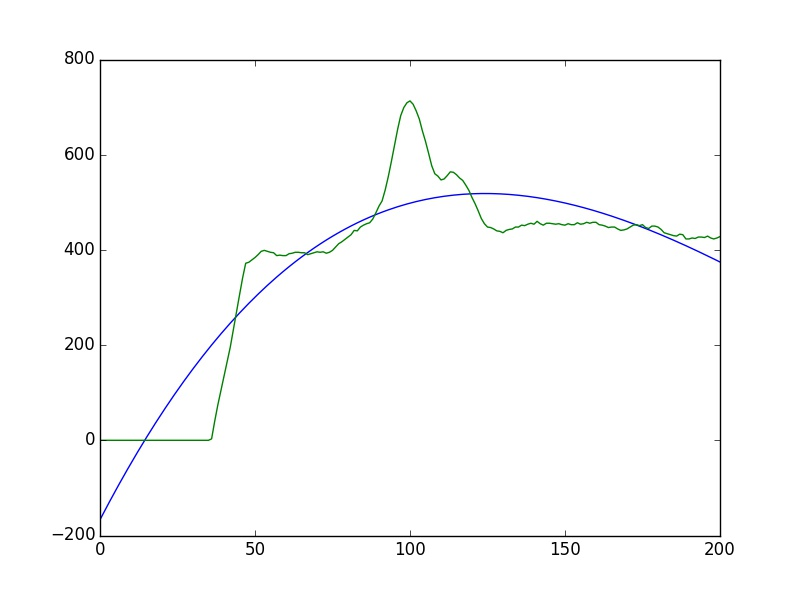于是想用高斯函数来实现对曲线的拟合，在matlab中试了下，三阶高斯拟合可以很好的拟合曲线，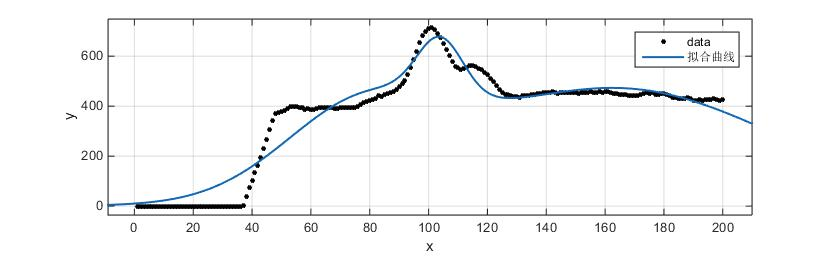但是numpy以及sicpy中没有找到类似poly1d这种对象，虽然可以自己定义高斯函数，如下
    def gaussian(self, x, *param):
fun = param*np.exp(-np.power(x - param, 2.) / (2 * np.power(param,        2.)))+param*np.exp(-np.power(x - param, 2.) / (2 * np.power(param, 2.)))
return fun
但是，在通过最小二乘拟合得到函数参数后只能得到拟合后的点，无法直接求导数..所以并不适合。
所以还是只能回到多项式拟合，如果4阶多项式不能表征的话，更高阶的呢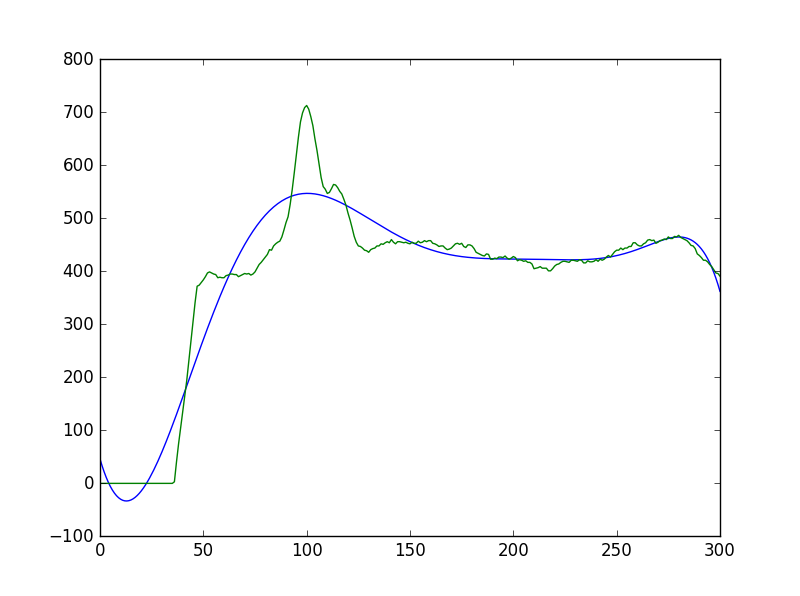总体来说，效果还是可以接受的。
如果下阶段找到好的高斯函数拟合方法，会继续更新。
展开全文python
• 这些只是一些快速肮脏的建议....如果您具有符号功能,无法分析计算导数,您可以随时评估一组点上的函数,并使用本页列出的其他方法来评估导数.>在大多数情况下,您有一组点(xi,fi),并且必须使用以下方法之一…....

这些只是一些快速肮脏的建议.希望有人会发现他们有帮助！
你有符号功能还是一组点？
>如果你有一个符号功能,你可能可以分析地计算导数. (可能的话,如果这样做很简单,你就会做到这一点,你不会在这里寻找替代品.)
>如果您具有符号功能,无法分析计算导数,您可以随时评估一组点上的函数,并使用本页列出的其他方法来评估导数.
>在大多数情况下,您有一组点(xi,fi),并且必须使用以下方法之一….
你的网格是平均的还是不均匀的？
>如果您的网格间隔均匀,您可能需要使用有限差分方案(参见维基百科文章here或here),除非您使用周期性边界条件(见下文). Here是在网格上求解普通微分方程的上下文中有限差分法的一个体面的介绍(特别是幻灯片9-14).这些方法通常在计算上是有效的,易于实现,并且该方法的误差可以被简单地估计为用于导出它的泰勒展开的截断误差.
>如果您的网格不均匀,您仍然可以使用有限差分方案,但是表达式更加困难,并且精度随着网格的均匀程度而非常强烈地变化.如果您的网格非常不均匀,您可能需要使用大的模具尺寸(更多相邻点)来计算给定点处的导数.人们经常构造内插多项式(通常为Lagrange polynomial),并区分该多项式以计算导数.参见例如this StackExchange问​​题.通过使用这些方法来估计错误通常很困难(尽管有些尝试这样做：here和here).在这些情况下,Fornberg’s method通常非常有用….
>必须注意您的域的边界,因为模板通常涉及域外的点.有些人引入“鬼点”,或者将边界条件与不同顺序的导数组合起来,以消除这些“鬼点”并简化模板.另一种方法是使用右侧或左侧的有限差分法.
> Here’s是有限差分方法的优秀“作弊表”,包括低阶中心,左右方式.我在工作站附近保存打印输出,因为我发现它很有用.
你的域定期吗？你能假定周期边界条件吗？
>如果您的域是周期性的,您可以使用傅立叶光谱方法计算导数到非常高的精度.这种技术有一些牺牲性能以获得高精度.事实上,如果你使用N点,那么你对衍生的估计大概是N ^ th的准确度.有关更多信息,请参阅(例如)this WikiBook.
傅立叶方法通常使用快速傅里叶变换(FFT)算法来实现大致O(N log(N))性能,而不是天真实现的离散傅里叶变换(DFT)可能采用的O(N ^ 2)算法.
>如果您的功能和域不是周期性的,则不应使用傅立叶光谱方法.如果您尝试使用不是周期性的功能,您将收到较大的错误和不良的“振铃”现象.
>任何阶数的计算导数需要1)从网格空间到谱空间的变换(O(N log(N))),2)傅里叶系数乘以它们的光谱波数(O(N)),以及2)从频谱空间到网格空间的反向变换(再次为O(N log(N))).
>将傅里叶系数乘以其光谱波数时必须小心. FFT算法的每个实现似乎都有自己的频谱模式和归一化参数的排序.例如,参见Math StackExchange上的this question的答案,有关在MATLAB中执行此操作的注意事项.
你正在寻找什么级别的准确性？您是否需要在给定的公差范围内计算衍生物？
>出于许多目的,一阶或二阶有限差分方案可能就足够了.为了获得更高的精度,您可以使用更高阶的泰勒扩展,降低高阶项.
>如果您需要在给定的公差范围内计算衍生工具,您可能需要查找具有所需错误的高阶方案.
通常,减少误差的最佳方法是在有限差分方案中减少网格间距,但这并不总是可能的.
>请注意,高阶有限差分方案几乎总是需要较大的模板尺寸(更邻近点).这可能会导致边界的问题. (见上面关于鬼点的讨论)
5.您对衍生工具是否与您的功能定义相同点进行评估是否重要？
>在大多数情况下,我们希望在所有点评估导数,这意味着我们要使用diff方法以外的东西.
你需要计算多个衍生品的订单吗？
>可以设置一个方程组,其中网格点函数值和这些点上的一阶和二阶导数都依赖于彼此.这可以通过在相邻点像往常一样组合泰勒展开,但保持衍生项而不是取消它们,并将它们与相邻点相连接.这些方程可以通过线性代数求解,不仅仅是一阶导数,而是第二类(或更高的顺序,如果设置正确).我相信这些被称为组合有限差分方案,它们通常与紧密有限差分方案结合使用,这将在下面讨论.
>目前的研究领域是如何最好地在紧凑型模具中解决多种衍生物.尽管许多研究人员倾向于针对特定需求(性能,准确性,稳定性或特定的流体动力学研究领域)来调整这些研究结果,结合紧凑的有限差分方法是有力且广泛适用的.
即将到来的例程
>我使用Fornberg的方法(见上文)开发一个小程序(nderiv_fornberg)来计算任意网格间距的一维维数.我觉得很容易使用.它使用边界上6点的双面模板和内部中心的5点模板.它可以在MATLAB文件交换here中找到.
结论
数值分化领域非常多样化.对于上面列出的每种方法,有许多变体具有自己的优点和缺点.这个职位几乎不是数字化差异的完全处理.
每个应用程序都不同希望这篇文章给感兴趣的读者一个有选择的考虑和资源的列表,以选择一种适合自己需要的方法.
可以通过代码片段和MATLAB特有的示例来改进此社区wiki.

展开全文• 参考文章：数学的凸凹性和拐点（数学知识：了解如何寻找曲线的拐点） 详细的:对函数求二阶导数，判断二阶导数的值，如果二阶导数为常数恒大于零-------------则在定义域内是上凹的，不存在拐点。
参考文章：数学的凸凹性和拐点（数学知识：了解如何寻找曲线的拐点）
详细的:对函数求二阶导数，判断二阶导数的值，
如果二阶导数为常数恒大于零-------------则在定义域内是上凹的，不存在拐点。
常数恒小于零------------则为下凹的，不存在拐点。
如果为多项式-----------则判断多项式等于零时，x的值，根据x值进行分段，判断每段的凹凸性。
遇到的问题：离散的值如何求拐点？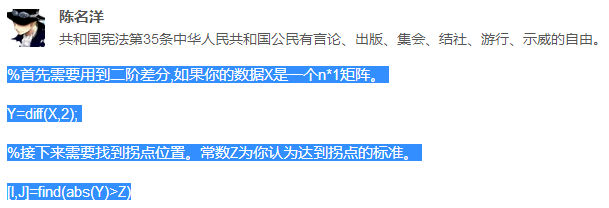Matlab中diff函数的用法

diff(X，2)是对函数X求二阶倒数，也就是用于求拐点和判断凹凸性的。

所做的实验：

我们首先设定函数定义域：

x∈[-10,10],步长为0.1

即程序：x=-10:0.1:10;

y=x.^3-12*x.^2;   % 因为是序列（矩阵),所以要加上个点

Y=diff(y,2);          %求二阶倒数

[I,J]=find(abs(Y)<0.001);  %找出二阶导数为零的在序列中的位置

实际上，我们通过计算，该函数的拐点为x=4,y=-128.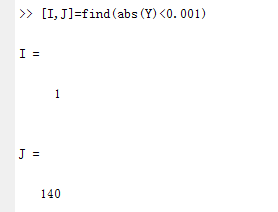x的值是这样求得的：x=-10+0.1*140=4.
则，y=4^3-12*4^2=-128.
结论是利用该方法求曲线的观点是可行的。


展开全文• MATLAB梯度和拉普拉斯算子在图像边缘检测中的应用数学方法边缘检测最通用的方法是检测灰度值的不连续性，这种不连续性用一阶和二阶导数来检测。（1）一阶导数：一阶导数即为梯度，对于平面上的图像来说，我们只需...卷积 边缘检测 计算机视觉
• diff、gradient 函数求拟合曲线的一、二阶导数 最后通过公式求得曲率 公式： 例：余弦函数取 8 个点，用 B-Spline 插值 x = 0:1:7; y = cos(x*0.5*pi); xx=0:0.01:7; yy=spline(x,y,xx); h1 = abs(diff(xx))...
• MATLAB算子在图像边缘检测中的应用数学方法边缘检测最通用的方法是检测灰度值的不连续性，这种不连续性用一阶和二阶导数来检测。（1）一阶导数：一阶导数即为梯度，对于平面上的图像来说，我们只需用到二维函数的...卷积 计算机视觉 边缘检测 深度学习 opencv
• 近几天没事，开始学习一下Matlab S函数求解二阶微分方程，具体方程表达式如下： D(q)q¨+C(q,q˙)q˙=τD\left( q \right)\ddot q + C(q,\dot q)\dot q = \tauD(q)q¨​+C(q,q˙​)q˙​=τ (2.1) τ=Kde˙+Kpe\tau ...
• MATLAB数据处理，针对x坐标不均匀离散点求导，二次求导后有较大波动，针对波动进行一些简单优化（非常规方法，仅具参考作用）。
• Preprocess代码  一. 求导 ...二阶导数MATLAB代码： X2st=diff(X,2); %X 为输入光谱矩阵，X2st 为输出二阶导数光谱矩阵   二. 多元散射校正 me=mean(X);  [m,~]=size(X); for i=1:m, p=p...高光谱 代码
• 边缘检测最通用的方法是检测灰度值的不连续性，这种不连续性使用一阶和二阶导数来检测。 图像处理中的二阶导数通常使用拉普拉斯算子来计算，二维函数f(x,y)的拉普拉斯算子由二阶微分构成: 拉普拉斯算子很少直接...
• 高斯函数的一阶、二阶导数也可以进行高通滤波，比如canny算子中用到的是高斯函数的一阶导数，LOG算子中用到的是高斯函数的二阶导数。高斯函数的相关公式如下所示： 　一维和二维高斯函数表达式分别为：　 　 　二...图像处理
• 时间离散化采用正向（或显式）欧拉方法，而空间二阶导数则采用二阶居中方案进行离散化。 Matlab脚本： Matlab实时脚本： 2.二维有限差分法（FD）的对流扩散方程 对流扩散方程使用有限差分法在二维矩形域上求解。 ...
• 时间离散化采用正向（或显式）欧拉方法，而空间二阶导数则采用二阶居中方案进行离散化。 Matlab脚本： Matlab实时脚本： 2.二维有限差分法（FD）的对流扩散方程 对流扩散方程使用有限差分法在二维矩形域上求解。 ...
• 此代码使用有限差分法求解平板上流动层流的相似方程... 我们在这里使用的控制变量是 eta（离散化间隔）和 alpha（速度剖面的二阶导数的斜率）。 参考书中详细介绍了离散化的推导：Adrian Bejan 的“对流热传递”。
• 方法 1 一阶梯度算子 检测赋值阈值，可检测边缘的方向 ...高斯滤波后再进行二阶导数，可先对高斯函数求二阶导数然后得到离散化模板，之后用模板和图像卷积 4 canny 以上在matlab中都采用edge实现。
•  因此求曲率的重点在于获得拟合曲线的一阶导数和二阶导数。 2 Matlab中的实现 2.1 实例1  根据参考资料的提示，可以使用csape()对离散点进行Spline插值，然后使用fnder()对得到Spline曲线求导，最后使用fnval()...
• 数值微分是用离散方法近似计算函数的导数值或偏导数值。...二阶导数的中心差商公式： 例1：利用数值微分求y=4*x^2+3*sin(x)在x=1处的近似导数。 先利用导函数求出其一阶和二阶
• ## Matlab学习——求解微分方程(组)

千次阅读 多人点赞 2018-05-29 17:19:00
1.在 Matlab 中，用大写字母 D 表示导数，Dy 表示 y 关于自变量的一阶导数，D2y 表示 y 关于自变量的二阶导数，依此类推.函数 dsolve 用来解决常微分方程（组）的求解问题，调用格式为 X=dsolve(‘eqn1’,’eqn2’...
• 主要参考学习《数字图像处理 原理与实践 matlab版》边缘检测的算法通常对领域内像素灰度求一阶导数、二阶导数以及梯度来实现，这些计算经过化简的结果成为算子（operator）。在使用算子进行边缘检测时，我们定义边缘...边缘检测
• = (dx/dt)^2+ x(t)(d^2x/dt^2) (1.1) % 在连续情况下（其中 x_ 表示 x 的一阶导数，x¨ 表示二阶导数%导数），并作为%[x[n]] = x^2[n] + x[n - 1]x[n + 1] (1.2) % 在离散情况下。 ％％ 方法%注意该函数被矢量化以...
• 在很多学科中的很多计算任务中都需要用到曲线的曲率（或者曲率半径），numpy库里和matlab build-in里都没有现成的能从离散点来算曲率的方法，网上找到的代码又不敢直接用，毕竟是要高频率用到自己科研上的工具，所以...
• 边缘检测的算法通常通过对邻域内像素灰度求一阶导数、二阶导数及梯度来实现，这些计算经过化简的结果称为算子。在计算时，由于灰度差分的结果通常较小，直接转换为灰度图会使检测结果模糊，可以使用阈值因子对差分...
• 更多阅读：sppy.site 背景 如何计算曲线 y(x) ~y(x)~ y(x) 上的曲率，而曲线是由若干离散点...和二阶导数 y′′ ~y''~ y′′ ，然后由下式计算 k=∣y′′∣(1+y′2)3/2...线性代数
• 一、拉普拉斯图像增强算法优化简介 图像Laplace变换是基本图像增强算法,原始图像通过Laplace变化后会增强图像中灰度突变处的...一个二维图像的Laplace算子是各项同性的二阶导数,定义为 该方程的离散形式为 图4是Lap
• 该处理器计算所述数据元素的至少一阶和/或二阶导数，并且计算该图像的等照度线曲率，所述曲率由κ标识。该处理器还确定校正因数α，该校正因数α对于由对象的曲率和/或所述数据的模糊造成的边缘错位进行校正。该校正......

# matlab离散二阶导数matlab 订阅